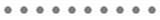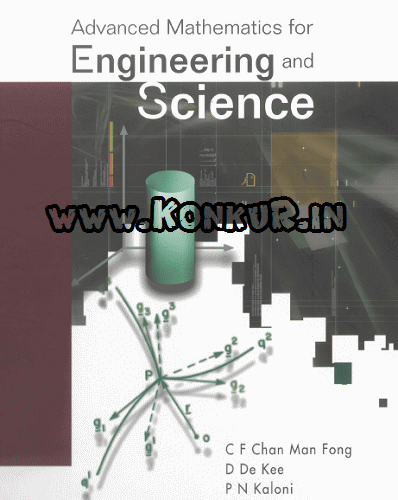# دانلود کتاب ریاضیات پیشرفته برای رشته های مهندسی و علوم پایه

• ۱۶ شهریور ۱۳۹۴
• بدون نظر
• 4,420 بار

دانلود کتاب ریاضیات پیشرفته برای رشته های مهندسی و علوم پایه

Advanced Mathematics for Engineering and Science

C F Chan Man Fong , D De Kee , P N KaloniThis is a mathematical text suitable for students of engineering and science who are at the third year undergraduate level or beyond. It is a book of applicable mathematics. It avoids the approach of listing only the techniques, followed by a few examples, without explaining why the techniques work. Thus, it provides not only the know-how but also the know-why. Equally, the text has not been written as a book of pure mathematics with a list of theorems followed by their proofs. The authors’ aim is to help students develop an understanding of mathematics and its applications. They have refrained from using clichés like “it is obvious” and “it can be shown”, which may be true only to a mature mathematician. On the whole, the authors have been generous in writing down all the steps in solving the example problems.

The book comprises ten chapters. Each chapter contains several solved problems clarifying the introduced concepts. Some of the examples are taken from the recent literature and serve to illustrate the applications in various fields of engineering and science. At the end of each chapter, there are assignment problems with two levels of difficulty. A list of references is provided at the end of the book

Contents
Review of Calculus and Ordinary Differential Equations
Series Solutions and Special Functions
Complex Variables
Vector and Tensor Analysis
Partial Differential Equations I
Partial Differential Equations II
Numerical Methods
Numerical Solution of Partial Differential Equations
Calculus of Variations
Special Topics

تعداد صفحات : 898
نویسندگان : C F Chan Man Fong , D De Kee , P N Kaloni
ویرایش : سال 2003
زبان : لاتین

• بر چسب ها :
• امیررضا توتچی اشتراک گذاری :عضویت
اطلاع از